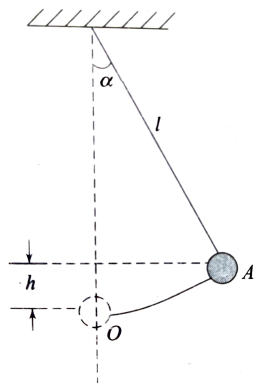Conservation of mechanical energy
Question

# A pendulum has a length l. Its bob is pulled aside from its equilibrium position through an angle $\mathrm{\alpha }$ and then released. The speed of the bob when it passes through the equilibrium position is given by

Moderate
Solution

## As shown in Fig., the height attained by the bob when the string subtends an angle $\mathrm{\alpha }$ with the vertical is$\mathrm{h}=\mathrm{l}-\mathrm{lcos}\mathrm{\alpha }=\mathrm{l}\left(1-\mathrm{cos}\mathrm{\alpha }\right)$Its potential energy at the highest point A = mgh, Where m is the mass of the bob. Let v be the speed of the bob when it passes through O. Its kinetic energy at $\mathrm{O}=\frac{1}{2}{\mathrm{mv}}^{2}$. From the principle of conservation of energy, we have$\frac{1}{2}{\mathrm{mv}}^{2}=\mathrm{mgh}$$\mathrm{v}=\sqrt{2\mathrm{gh}}=\sqrt{2\mathrm{gl}\left(1-\mathrm{cos\alpha }\right)}$Hence the correct choice is (c).

Get Instant Solutions